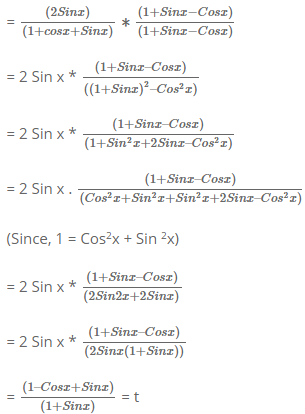Courses

# Test: Trigonometry- 1

## 4 Questions MCQ Test SSC CGL Tier 2 - Study Material, Online Tests, Previous Year | Test: Trigonometry- 1

Description
This mock test of Test: Trigonometry- 1 for Quant helps you for every Quant entrance exam. This contains 4 Multiple Choice Questions for Quant Test: Trigonometry- 1 (mcq) to study with solutions a complete question bank. The solved questions answers in this Test: Trigonometry- 1 quiz give you a good mix of easy questions and tough questions. Quant students definitely take this Test: Trigonometry- 1 exercise for a better result in the exam. You can find other Test: Trigonometry- 1 extra questions, long questions & short questions for Quant on EduRev as well by searching above.
QUESTION: 1

### If tanØ + sinØ = m, tanØ - sinØ = n, find the value of m2 - n2

Solution: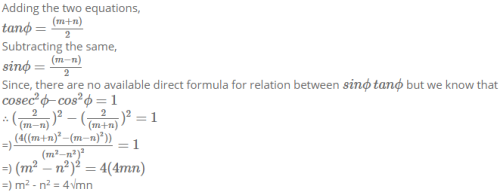QUESTION: 2

### A student is standing with a banner at the top of a 100 m high college building. From a point on the ground, the angle of elevation of the top of the student is 60° and from the same point, the angle of elevation of the top of the tower is 45°. Find the height of the student.

Solution: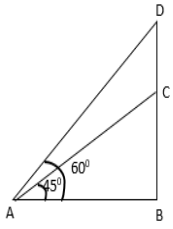Let BC be the height of the tower and DC be the height of the student.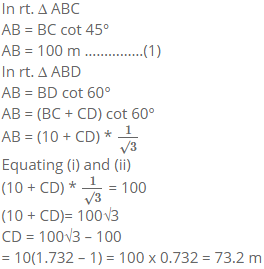QUESTION: 3

### If Cos x – Sin x = √2 Sin x, find the value of Cos x + Sin x:

Solution:

Cos x – Sin x = √2 Sin x

=) Cos x = Sin x + √2 Sin x

=) Cos x = Sin x + √2 Sin x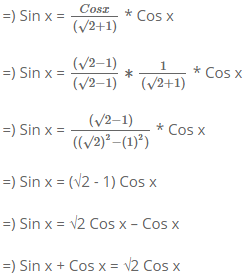QUESTION: 4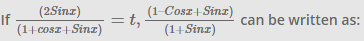Solution: